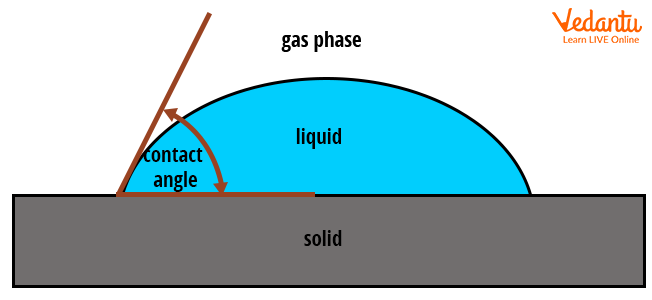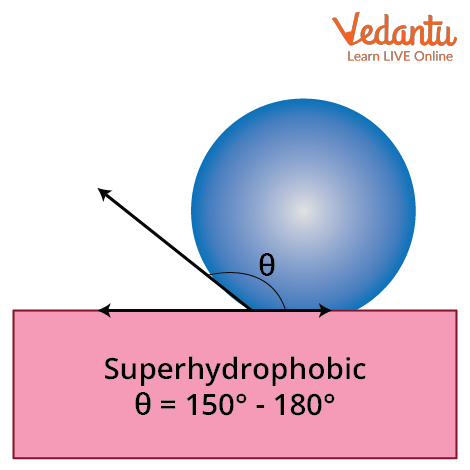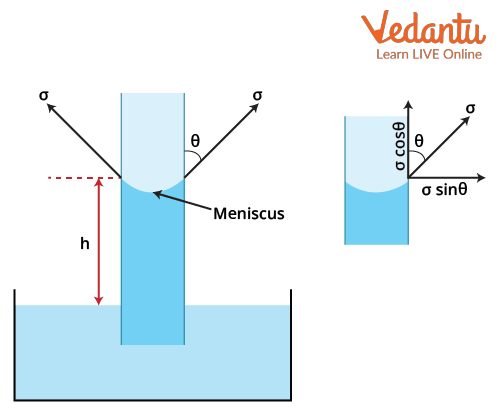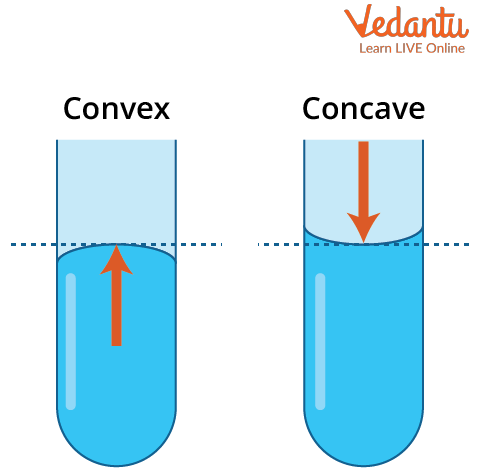Courses
Courses for Kids
Free study material
Offline Centres
More

# Surface Tension and Contact Angle for JEELast updated date: 28th Nov 2023
Total views: 20.4k
Views today: 0.20k## Contact Angle: Angle Between Surface and Liquid

When the fluid of the liquid gets transferred to other containers, it makes an angle of contact with the boundaries of the container. The contact happens because of the force of intermolecular attraction between the fluid and the solid surface which is known as ‘adhesion’. Similarly, the free surface of the fluid acts like a stretched membrane. Due to the intermolecular forces of attraction between the fluid particles, a force is developed on the surface which is known as ‘surface tension’.

In this article, an attempt has been made to give a better perspective and understanding of the given concepts (like contact angle, surface tension definition, angle and its measurement, angle of contact formula, angle of contact diagram and the zero angles of contact). It also covers a major section of surface tension and angle of contact in fluid mechanics.

## Cohesion

Cohesion is a surface property of the fluid which depicts the attraction forces between the same fluid particles towards each other. It represents the attraction forces between the molecules of the same fluid towards each other. For example, when mercury is split over a horizontal surface, the molecules of the mercury try to gather into droplets.

This tendency of mercury molecules to gather together is due to the cohesive nature of the fluid.  This condition of mercury gathering up into droplets and trying to stay away from the surface is called the ‘non-wetting condition’ and the fluid which follows this condition is known as ‘non-wetting fluid’.

Adhesion is the surface property of the fluid which depicts the force attraction between the molecules of different materials towards each other. In simple terms, the attraction of the forces occurs between the molecules of fluid and the solid surfaces towards each other. For example, when water is poured into a glass, the force of attraction occurs between water and the glass surface.

When water spills over the surface, it spreads over the surface. This condition is called the ‘wetting condition’ and the fluid that follows this condition is known as ‘wetting fluid’.

## What is the Angle of Contact?

The angle of contact is the angle made by the fluid surface with the horizontal surface. The angle of contact depends upon the nature of the fluid and the interface present between the surface and the fluid. The angle of contact is different for wetting fluid and non-wetting fluid. The angle of contact also depends upon the cleanliness of the interface between the fluid and the solid surface. The angle of the contact diagram of wetting and non-wetting fluid is given below:Angle of contact of a drop of liquid with the solid surface

• ### Hydrophilic

The contact angle for the wetting liquid is always less than 90o, which means that the force of attraction between the fluid particles and the liquid that tries to gather up into droplet form is due to the cohesive nature of the fluid. An example of wetting liquid is water.Image of the angle of contact for the wetting liquid

• ### Hydrophobic

The contact angle for non-wetting liquid is always greater than 90o , which means that the force of attraction that exists between the fluid particles and the horizontal surface is due to the adhesive nature of the fluid. This results in the spreading of water on the horizontal surface. An example of a non-wetting liquid is glycerin.Image of the angle of contact for the non-wetting liquid

• ### Ultrahydrophobic/ Superhydrophobic

Ultrahydrophobic or superhydrophobic are surfaces that are highly hydrophobic and they have contact angles of more than 150o. As can be guessed, these surfaces are extremely difficult to wet and this effect is also referred to as the lotus effect. This name is given because lotus leaves are superhydrophobic. If you’ve ever come across a lotus leaf, you might be familiar that a droplet striking its surface can fully rebound just like an elastic ball.Superhydrophobic surface

 Sr no. Surface wettability Name of the surface Contact Angle $\theta$ 1 Surface is wettable Hydrophilic 0o < $\theta$ < 90o 2 Surface is not wettable Hydrophobic 90o < $\theta$ < 150o 3 Surface is liquid repellent Superhydrophobic 150o < $\theta$ < 180o

## Meniscus Effect

The meniscus effect is the phenomenon of liquid rising or falling in a capillary tube. When the capillary is dipped into a water-containing container, the level of water that rises is more in the tube than in the container. That rise happens due to capillary action. It occurs due to the intermolecular force of attraction between the liquid and the tube surface.

When water is used as the liquid, the adhesion between the liquid surface and the tube surface is greater than the cohesion between the water molecules. Therefore, a tangent forming from the surface of the water in a tube makes an angle of contact with the tube surface. Since this angle is less than $90^{\circ}$, a concave cavity is formed.

The angle of contact formula is derived with the help of the concept of the meniscus effect.Meniscus effect

The weight of the liquid raised will be equal to the vertical component of the surface tension force of the fluid.

Weight of the liquid raised =(area of tube $\times$ rising or falling in height) $\times$ specific weight

$=\left(\dfrac{\pi}{4} d^{2} h\right) \times w$

Vertical component of surface tension force $=\sigma \cos \theta \times$ circumference

$=\sigma \cos \theta \times \pi d$

Weight of the liquid raised = Vertical component of surface tension force

\begin{align} \left(\dfrac{\pi}{4} d^{2} h\right) \times w &=\sigma \cos \theta \pi d \\ \cos \theta &=\dfrac{h w d}{4 \sigma} \\ \theta &=\cos ^{-1}\left(\dfrac{h w d}{4 \sigma}\right) \end{align}

The above relation is the angle of contact formula.

• When the value of angle lies in the given range $0 \leq \theta < 90^{\circ}$, a concave meniscus will form.

• When the value of the angle of contact lies in the given range $90^{\circ} \leq \theta < 180^{\circ}$, a convex meniscus will form.

The condition for zero angles of contact happens when the tangent of surface tension force will become collinear or parallel with the side surface of the capillary tube.Convex and concave meniscus

## Surface Tension Definition

Surface tension is the property of the fluid that acts on the surface of the liquid and seems like a stretched membrane. The fluid tends to shrink into the minimum possible area of the liquid. In simple terms, surface tension is the tension force that occurs at the surface film of the liquid, which is caused by the attraction of the fluid particles. This force tends to minimize the surface area of the fluid.

Surface tension is defined as the energy expended per unit of the surface of a fluid. Mathematically, it is written as:

Surface tension $=\dfrac{\text { Energy }}{\text { Area }}$

$\sigma=\dfrac{E}{A}$

$\sigma=$ Surface tension

E = Energy expended by the surface of the fluid

A = Surface of fluid

The dimension formula for the surface tension is derived by substituting the dimensional formula of energy and area in the above relationship. Mathematically, it is written as:

• The dimensional formula of energy is, $E=\left(M^{1} L^{2} T^{-2}\right)$

• The dimensional formula of the area is, $A=\left(M^{0} L^{2} T^{0}\right)$

Therefore, the dimensional formula for surface tension will be,

\begin{align} &\sigma=\dfrac{E}{A} \\ \\ &\sigma=\dfrac{\left(M^{1} L^{2} T^{-2}\right)}{\left(M^{0} L^{2} T^{0}\right)} \\ \\ &\sigma=\dfrac{\left(M^{1} L^{2} T^{-2}\right)}{\left(L^{2}\right)} \\ &\sigma=\left(M^{1} T^{-2}\right) \end{align}

Hence, the dimension formula for surface tension is (M1T-2).

## Conclusion

This article concludes with qualitative information about the angle of contact and the cohesive and adhesive nature of the fluid. It also describes the condition of the fluid in wetting and non-wetting conditions. It further explained the value of the angle of contact in wetting and non-wetting conditions of the fluid. This article attempted to cover all queries (definition of angle of contact, explanation of surface tension and angle of contact, zero angles of contact, etc.) and tried to give better clarity to the concept.

## FAQs on Surface Tension and Contact Angle for JEE

1. What is the weightage of this section in the competitive JEE exam?

The weightage of this section is 2-5% according to the JEE point of view. This section comes in the category of fluid mechanics. This concept was found to be the most important topic as per the JEE perspective. This concept has a variety of numerical related to fluid mechanics, which enhances its importance in the JEE exam. Various other important things related to fluid mechanics are surface tension, the flow of fluid in pipes, the concept of the Bernoulli equation, and the Bernoulli principle.

2. What are the factors which can increase surface tension?

One of the primary factors which can increase the effect on the surface tension of the fluid is temperature. Whenever there is a change in the value of temperature, the surface tension of the fluid changes. The relation of surface tension of fluid with temperature is indirectly proportional to each other. As the temperature increases, the surface tension of the fluid decreases. If the temperature decreases, then the surface tension of the fluid also increases.

3. What are the factors on which the angle of contact depends?

The angle of contact which is made by the fluid and the surface placed in contact purely depends upon the medium of the free surface of the liquid, the solid surface which is in contact with the fluid, the nature of the liquid and the cleanliness of the liquid-solid interface. All of these factors play a major role in affecting the angle of contact of the fluid with the solid surface. One of the most important things is that the angle of contact also changes with the temperature and the purity of the fluid.